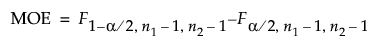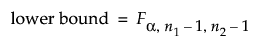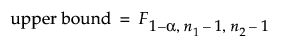Design of Experiments Guide > Sample Size Explorers > Confidence Interval Calculators > Margin of Error for Two Independent Sample Variances
Publication date: 11/10/2021

## Margin of Error for Two Independent Sample Variances

Use the Interval Explorer for Two Independent Sample Variance to determine a sample size for a confidence interval. Select DOE > Sample Size Explorers > Confidence Intervals > Margin of Error for Two Independent Sample Variances. Explore the trade offs between sample size, significance, and the margin of error for your interval.

### Interval Explorer for Two Independent Sample Variances Options

Set study assumptions and explore sample sizes using the radio buttons, text boxes, and sliders. The curve updates as you make changes to the settings. Alternatively, change settings by dragging the cross hairs on the curve or adjusting the values in the axis text boxes.

Interval Type

Lower Bound

Specifies a one-sided lower interval.

Upper Bound

Specifies a one-sided upper interval.

Interval

Specifies a two-sided interval.

Fixed Parameters

Alpha

Specifies the confidence level, 1 - Alpha. The default alpha level is 0.05 for a 95% confidence interval.

Interval Parameters

Group 1 Sample Size

Specifies the number of observations (runs, experimental units, or samples) needed for Group 1 in your experiment. Select Lock to lock the Group 1 sample size.

Group 2 Sample Size

Specifies the number of observations (runs, experimental units, or samples) needed for Group 2 in your experiment. Select Lock to lock the Group 2 sample size.

Total Sample Size

Specifies the total number of observations (runs, experimental units, or samples) needed for your experiment. The margin of error curve is based on total sample size. Select Lock to lock the total sample size.

Note: Adjusting the sample size for one group adjusts the total sample size unless the total sample size is locked. In that case, adjusting the sample size for one group adjust the sample size for the second group. Adjusting the total sample size adjusts the two groups proportionally, unless one group is locked. Use only one lock at a time.

Interval Width

Specifies the full width of the interval. With all other parameters fixed, interval width decreases as sample size increases.

Save Settings

Saves the current settings to the Saved Settings table. This enables you to save a set of alternative study plans. See Saved Settings in the Sample Size Explorers.

Make Data Collection Table

Creates a new data table that you can use for data collection.

### Statistical Details for the Ratio Two Independent Variances Interval Explorer

The interval calculations for capturing the ratio of two population variances is based on the F-distribution. The margin of error (MOE) is calculated as follows:The lower bound is calculated as follows:The upper bound is calculated as follows: